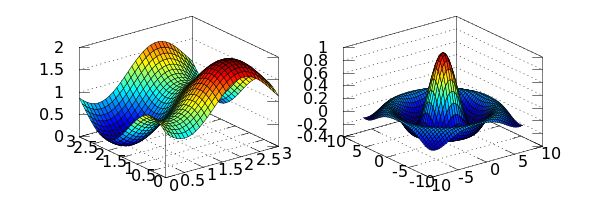# The cell magics in IPython¶

IPython has a system of commands we call 'magics' that provide effectively a mini command language that is orthogonal to the syntax of Python and is extensible by the user with new commands. Magics are meant to be typed interactively, so they use command-line conventions, such as using whitespace for separating arguments, dashes for options and other conventions typical of a command-line environment.

Magics come in two kinds:

• Line magics: these are commands prepended by one % character and whose arguments only extend to the end of the current line.
• Cell magics: these use two percent characters as a marker (%%), and they receive as argument both the current line where they are declared and the whole body of the cell. Note that cell magics can only be used as the first line in a cell, and as a general principle they can't be 'stacked' (i.e. you can only use one cell magic per cell). A few of them, because of how they operate, can be stacked, but that is something you will discover on a case by case basis.

The %lsmagic magic is used to list all available magics, and it will show both line and cell magics currently defined:

In :
%lsmagic

Out:
Available line magics:
%alias  %alias_magic  %autocall  %automagic  %autosave  %bookmark  %cd  %clear  %colors  %config  %connect_info  %debug  %dhist  %dirs  %doctest_mode  %ed  %edit  %env  %gist  %gui  %hist  %history  %install_default_config  %install_ext  %install_profiles  %killbgscripts  %less  %load  %load_ext  %loadpy  %logoff  %logon  %logstart  %logstate  %logstop  %lsmagic  %macro  %magic  %man  %matplotlib  %more  %notebook  %page  %pastebin  %pdb  %pdef  %pdoc  %pfile  %pinfo  %pinfo2  %popd  %pprint  %precision  %profile  %prun  %psearch  %psource  %pushd  %pwd  %pycat  %pylab  %qtconsole  %quickref  %recall  %rehashx  %reload_ext  %rep  %rerun  %reset  %reset_selective  %run  %save  %sc  %store  %sx  %system  %tb  %tic  %time  %timeit  %toc  %unalias  %unload_ext  %who  %who_ls  %whos  %xdel  %xmode

Available cell magics:
%%!  %%HTML  %%SVG  %%bash  %%capture  %%debug  %%file  %%html  %%javascript  %%latex  %%perl  %%prun  %%pypy  %%python  %%python3  %%ruby  %%script  %%sh  %%svg  %%sx  %%system  %%time  %%timeit  %%writefile

Automagic is ON, % prefix IS NOT needed for line magics.

Since in the introductory section we already covered the most frequently used line magics, we will focus here on the cell magics, which offer a great amount of power.

Let's load matplotlib and numpy so we can use numerics/plotting at will later on.

In :
%matplotlib inline
import numpy as np
import matplotlib.pyplot as plt


## Some simple cell magics¶

Timing the execution of code; the 'timeit' magic exists both in line and cell form:

In :
%timeit np.linalg.eigvals(np.random.rand(100,100))

100 loops, best of 3: 6.05 ms per loop

In :
%%timeit a = np.random.rand(100, 100)
np.linalg.eigvals(a)

100 loops, best of 3: 6.07 ms per loop


The %%capture magic can be used to capture the stdout/err of any block of python code, either to discard it (if it's noise to you) or to store it in a variable for later use:

In :
%%capture capt
from __future__ import print_function
import sys
print('Hello stdout')
print('and stderr', file=sys.stderr)

In :
capt.stdout, capt.stderr

Out:
('Hello stdout\n', 'and stderr\n')
In :
capt.show()

Hello stdout

and stderr


The %%writefile magic is a very useful tool that writes the cell contents as a named file:

In :
%%writefile foo.py
print('Hello world')

Writing foo.py

In :
%run foo

Hello world


## Magics for running code under other interpreters¶

IPython has a %%script cell magic, which lets you run a cell in a subprocess of any interpreter on your system, such as: bash, ruby, perl, zsh, R, etc.

It can even be a script of your own, which expects input on stdin.

To use it, simply pass a path or shell command to the program you want to run on the %%script line, and the rest of the cell will be run by that script, and stdout/err from the subprocess are captured and displayed.

In :
%%script python
import sys
print 'hello from Python %s' % sys.version

hello from Python 2.7.2 (default, Oct 11 2012, 20:14:37)
[GCC 4.2.1 Compatible Apple Clang 4.0 (tags/Apple/clang-418.0.60)]

In :
%%script python3
import sys
print('hello from Python: %s' % sys.version)

hello from Python: 3.3.1 (v3.3.1:d9893d13c628, Apr  6 2013, 11:07:11)
[GCC 4.2.1 (Apple Inc. build 5666) (dot 3)]


IPython also creates aliases for a few common interpreters, such as bash, ruby, perl, etc.

These are all equivalent to %%script <name>

In :
%%ruby
puts "Hello from Ruby #{RUBY_VERSION}"

Hello from Ruby 1.9.3

In :
%%bash
echo "hello from $BASH"  hello from /usr/local/bin/bash  ## Exercise: write your own script that numbers input lines¶ Write a file, called lnum.py, such that the following cell works as shown (hint: don't forget about the executable bit!): In : %%script ./lnum.py my first line my second more  0: my first line 1: my second 2: more ---- END ----  ## Capturing output¶ You can also capture stdout/err from these subprocesses into Python variables, instead of letting them go directly to stdout/err In : %%bash echo "hi, stdout" echo "hello, stderr" >&2  hi, stdout  hello, stderr  In : %%bash --out output --err error echo "hi, stdout" echo "hello, stderr" >&2  In : print(error) print(output)  hello, stderr hi, stdout  ## Background Scripts¶ These scripts can be run in the background, by adding the --bg flag. When you do this, output is discarded unless you use the --out/err flags to store output as above. In : %%ruby --bg --out ruby_lines for n in 1...10 sleep 1 puts "line #{n}" STDOUT.flush end  Starting job # 0 in a separate thread.  When you do store output of a background thread, these are the stdout/err pipes, rather than the text of the output. In : ruby_lines  Out: <open file '<fdopen>', mode 'rb' at 0x112cd55d0> In : print(ruby_lines.read())  line 1 line 2 line 3 line 4 line 5 line 6 line 7 line 8 line 9  # Cython Magic Functions Extension¶ ## Loading the extension¶ IPtyhon has a cythonmagic extension that contains a number of magic functions for working with Cython code. This extension can be loaded using the %load_ext magic as follows: In : %load_ext cythonmagic  The %%cython_pyximport magic allows you to enter arbitrary Cython code into a cell. That Cython code is written as a .pyx file in the current working directory and then imported using pyximport. You have the specify the name of the module that the Code will appear in. All symbols from the module are imported automatically by the magic function. In : %%cython_pyximport foo def f(x): return 4.0*x  In : f(10)  Out: 40.0 ## The %cython magic¶ Probably the most important magic is the %cython magic. This is similar to the %%cython_pyximport magic, but doesn't require you to specify a module name. Instead, the %%cython magic uses manages everything using temporary files in the ~/.cython/magic directory. All of the symbols in the Cython module are imported automatically by the magic. Here is a simple example of a Black-Scholes options pricing algorithm written in Cython: In : %%cython cimport cython from libc.math cimport exp, sqrt, pow, log, erf @cython.cdivision(True) cdef double std_norm_cdf(double x) nogil: return 0.5*(1+erf(x/sqrt(2.0))) @cython.cdivision(True) def black_scholes(double s, double k, double t, double v, double rf, double div, double cp): """Price an option using the Black-Scholes model. s : initial stock price k : strike price t : expiration time v : volatility rf : risk-free rate div : dividend cp : +1/-1 for call/put """ cdef double d1, d2, optprice with nogil: d1 = (log(s/k)+(rf-div+0.5*pow(v,2))*t)/(v*sqrt(t)) d2 = d1 - v*sqrt(t) optprice = cp*s*exp(-div*t)*std_norm_cdf(cp*d1) - \ cp*k*exp(-rf*t)*std_norm_cdf(cp*d2) return optprice  In : black_scholes(100.0, 100.0, 1.0, 0.3, 0.03, 0.0, -1)  Out: 10.327861752731728 In : %timeit black_scholes(100.0, 100.0, 1.0, 0.3, 0.03, 0.0, -1)  1000000 loops, best of 3: 366 ns per loop  Cython allows you to specify additional libraries to be linked with your extension, you can do so with the -l flag (also spelled --lib). Note that this flag can be passed more than once to specify multiple libraries, such as -lm -llib2 --lib lib3. Here's a simple example of how to access the system math library: In : %%cython -lm from libc.math cimport sin print 'sin(1)=', sin(1)  sin(1)= 0.841470984808  You can similarly use the -I/--include flag to add include directories to the search path, and -c/--compile-args to add extra flags that are passed to Cython via the extra_compile_args of the distutils Extension class. Please see the Cython docs on C library usage for more details on the use of these flags. # Rmagic Functions Extension¶ IPython has an rmagic extension that contains a some magic functions for working with R via rpy2. This extension can be loaded using the %load_ext magic as follows: In : %load_ext rmagic  A typical use case one imagines is having some numpy arrays, wanting to compute some statistics of interest on these arrays and return the result back to python. Let's suppose we just want to fit a simple linear model to a scatterplot. In : %matplotlib inline import numpy as np import matplotlib.pyplot as plt  In : X = np.array([0,1,2,3,4]) Y = np.array([3,5,4,6,7]) plt.scatter(X, Y)  Out: <matplotlib.collections.PathCollection at 0x112ab1f90>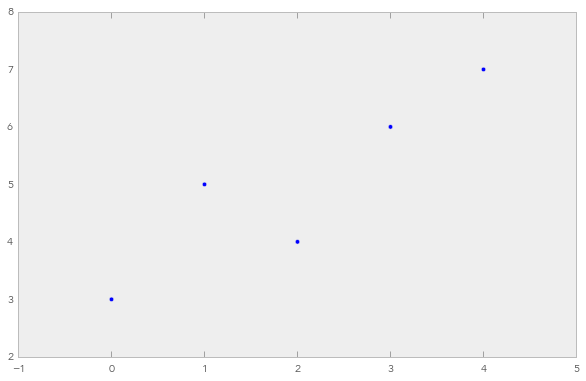We can accomplish this by first pushing variables to R, fitting a model and returning the results. The line magic %Rpush copies its arguments to variables of the same name in rpy2. The %R line magic evaluates the string in rpy2 and returns the results. In this case, the coefficients of a linear model. In : %Rpush X Y %R lm(Y~X)$coef

Out:
array([ 3.2,  0.9])

It is also possible to return more than one value with %R.

In :
%R resid(lm(Y~X)); coef(lm(X~Y))

Out:
array([-2.5,  0.9])

One can also easily capture the results of %R into python objects. Like R, the return value of this multiline expression (multiline in the sense that it is separated by ';') is the final value, which is the coef(lm(X~Y)). To pull other variables from R, there is one more magic.

There are two more line magics, %Rpull and %Rget. Both are useful after some R code has been executed and there are variables in the rpy2 namespace that one would like to retrieve. The main difference is that one returns the value (%Rget), while the other pulls it to self.shell.user_ns (%Rpull). Imagine we've stored the results of some calculation in the variable "a" in rpy2's namespace. By using the %R magic, we can obtain these results and store them in b. We can also pull them directly to user_ns with %Rpull. They are both views on the same data.

In :
b = %R a=resid(lm(Y~X))
%Rpull a
print(a)
assert id(b.data) == id(a.data)
%R -o a

[-0.2  0.9 -1.   0.1  0.2]


## Plotting and capturing output¶

R's console (i.e. its stdout() connection) is captured by ipython, as are any plots which are published as PNG files like the notebook with arguments --pylab inline. As a call to %R may produce a return value (see above) we must ask what happens to a magic like the one below. The R code specifies that something is published to the notebook. If anything is published to the notebook, that call to %R returns None.

In :
from __future__ import print_function
v1 = %R plot(X,Y); print(summary(lm(Y~X))); vv=mean(X)*mean(Y)
print('v1 is:', v1)
v2 = %R mean(X)*mean(Y)
print('v2 is:', v2)

Call:
lm(formula = Y ~ X)

Residuals:
1    2    3    4    5
-0.2  0.9 -1.0  0.1  0.2

Coefficients:
Estimate Std. Error t value Pr(>|t|)
(Intercept)   3.2000     0.6164   5.191   0.0139 *
X             0.9000     0.2517   3.576   0.0374 *
---
Signif. codes:  0 ‘***’ 0.001 ‘**’ 0.01 ‘*’ 0.05 ‘.’ 0.1 ‘ ’ 1

Residual standard error: 0.7958 on 3 degrees of freedom
Multiple R-squared:   0.81,	Adjusted R-squared:  0.7467
F-statistic: 12.79 on 1 and 3 DF,  p-value: 0.03739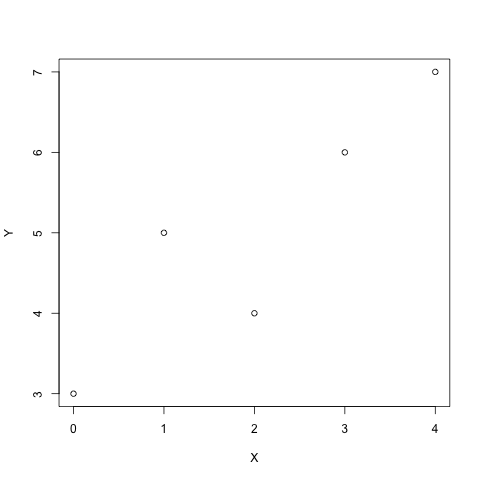v1 is: [ 10.]
v2 is: [ 10.]


## Cell level magic¶

Often, we will want to do more than a simple linear regression model. There may be several lines of R code that we want to use before returning to python. This is the cell-level magic.

For the cell level magic, inputs can be passed via the -i or --inputs argument in the line. These variables are copied from the shell namespace to R's namespace using rpy2.robjects.r.assign. It would be nice not to have to copy these into R: rnumpy ( http://bitbucket.org/njs/rnumpy/wiki/API ) has done some work to limit or at least make transparent the number of copies of an array. This seems like a natural thing to try to build on. Arrays can be output from R via the -o or --outputs argument in the line. All other arguments are sent to R's png function, which is the graphics device used to create the plots.

We can redo the above calculations in one ipython cell. We might also want to add some output such as a summary from R or perhaps the standard plotting diagnostics of the lm.

In :
%%R -i X,Y -o XYcoef
XYlm = lm(Y~X)
XYcoef = coef(XYlm)
print(summary(XYlm))
par(mfrow=c(2,2))
plot(XYlm)

Call:
lm(formula = Y ~ X)

Residuals:
1    2    3    4    5
-0.2  0.9 -1.0  0.1  0.2

Coefficients:
Estimate Std. Error t value Pr(>|t|)
(Intercept)   3.2000     0.6164   5.191   0.0139 *
X             0.9000     0.2517   3.576   0.0374 *
---
Signif. codes:  0 ‘***’ 0.001 ‘**’ 0.01 ‘*’ 0.05 ‘.’ 0.1 ‘ ’ 1

Residual standard error: 0.7958 on 3 degrees of freedom
Multiple R-squared:   0.81,	Adjusted R-squared:  0.7467
F-statistic: 12.79 on 1 and 3 DF,  p-value: 0.03739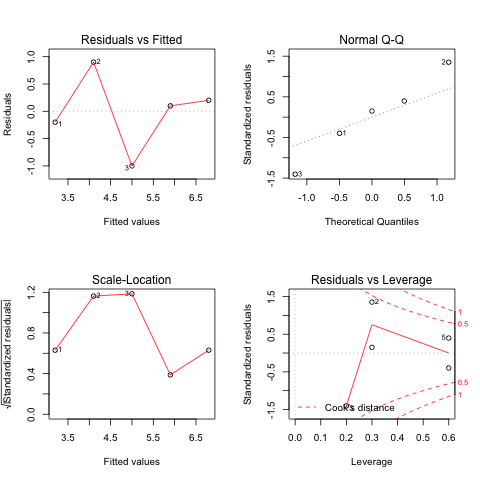# octavemagic: Octave inside IPython¶

The octavemagic extension provides the ability to interact with Octave. It depends on the oct2py and h5py packages, which may be installed using easy_install. It has been closely modeled after the R extension, so many of its names and usage patterns are the same.

To enable the extension, load it as follows:

In :
%load_ext octavemagic


## Overview¶

Loading the extension enables three magic functions: %octave, %octave_push, and %octave_pull.

The first is for executing one or more lines of Octave, while the latter allow moving variables between the Octave and Python workspace. Here you see an example of how to execute a single line of Octave, and how to transfer the generated value back to Python:

In :
x = %octave [1 2; 3 4];
x

Out:
array([[ 1.,  2.],
[ 3.,  4.]])
In :
a = [1, 2, 3]

%octave_push a
%octave a = a * 2;
%octave_pull a
a

Out:
array([[2, 4, 6]])

When using the cell magic, %%octave (note the double %), multiple lines of Octave can be executed together. Unlike with the single cell magic, no value is returned, so we use the -i and -o flags to specify input and output variables.

In :
%%octave -i x -o y
y = x + 3;

In :
y

Out:
array([[ 4.,  5.],
[ 6.,  7.]])

## Plotting¶

Plot output is automatically captured and displayed, and using the -f flag you may choose its format (currently, png and svg are supported).

In :
%%octave -f svg

p = [12 -2.5 -8 -0.1 8];
x = 0:0.01:1;

polyout(p, 'x')
plot(x, polyval(p, x));

12*x^4 - 2.5*x^3 - 8*x^2 - 0.1*x^1 + 8

The plot size is adjusted using the -s flag:

In :
%%octave -s 500,500

# butterworth filter, order 2, cutoff pi/2 radians
b = [0.292893218813452  0.585786437626905  0.292893218813452];
a = [1  0  0.171572875253810];
freqz(b, a, 32);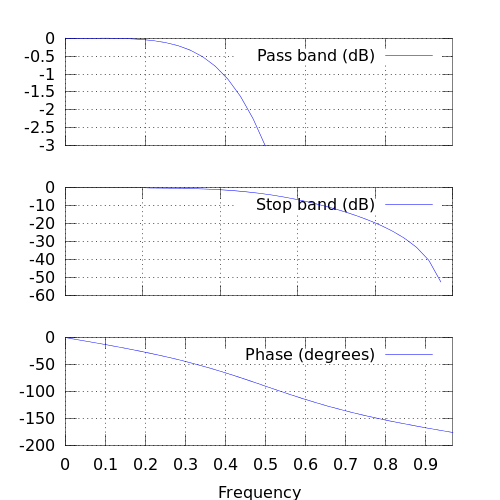In :
%%octave -s 600,200 -f png

subplot(121);
[x, y] = meshgrid(0:0.1:3);
r = sin(x - 0.5).^2 + cos(y - 0.5).^2;
surf(x, y, r);

subplot(122);
sombrero()